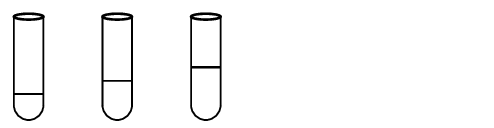Home Practice
For learners and parents For teachers and schools
Textbooks
Full catalogue
Pricing SupportLog in

We think you are located in United States. Is this correct?

# 13.3 Law of constant composition

## 13.3 Law of constant composition (ESADW)

In any given chemical compound, the elements always combine in the same proportion with each other. This is the law of constant composition.

The law of constant composition says that, in any particular chemical compound, all samples of that compound will be made up of the same elements in the same proportion or ratio. For example, any water molecule is always made up of two hydrogen atoms and one oxygen atom in a $$2:1$$ ratio. If we look at the relative masses of oxygen and hydrogen in a water molecule, we see that $$\text{94}\%$$ of the mass of a water molecule is accounted for by oxygen and the remaining $$\text{6}\%$$ is the mass of hydrogen. This mass proportion will be the same for any water molecule.

This does not mean that hydrogen and oxygen always combine in a $$2:1$$ ratio to form $$\text{H}_{2}\text{O}$$. Multiple proportions are possible. For example, hydrogen and oxygen may combine in different proportions to form $$\text{H}_{2}\text{O}_{2}$$ rather than $$\text{H}_{2}\text{O}$$. In $$\text{H}_{2}\text{O}_{2}$$, the $$\text{H}:\text{O}$$ ratio is $$1:1$$ and the mass ratio of hydrogen to oxygen is $$1:16$$. This will be the same for any molecule of hydrogen peroxide.

## Law of constant composition

### Aim

To investigate the ratio in which compounds combine.

### Apparatus

• $$\text{0,1}$$ $$\text{mol·dm^{-3}}$$ silver nitrate ($$\text{AgNO}_{3}$$)

• $$\text{0,1}$$ $$\text{mol·dm^{-3}}$$ sodium chloride ($$\text{NaCl}$$)

• $$\text{0,1}$$ $$\text{mol·dm^{-3}}$$ lead nitrate ($$\text{PbNO}_{3}$$)

• $$\text{0,1}$$ $$\text{mol·dm^{-3}}$$ sodium iodide ($$\text{NaI}$$)

• $$\text{0,1}$$ $$\text{mol·dm^{-3}}$$ iron (III) chloride ($$\text{FeCl}_{3}$$)

• $$\text{0,1}$$ $$\text{mol·dm^{-3}}$$ sodium hydroxide ($$\text{NaOH}$$)

• 9 large test tubes

• 3 propettes### Method

Reaction 1: Prepare three test tubes with $$\text{5}$$ $$\text{mL}$$, $$\text{10}$$ $$\text{mL}$$ and $$\text{15}$$ $$\text{mL}$$ of silver nitrate respectively. Using a clean propette add $$\text{5}$$ $$\text{mL}$$ of sodium chloride to each one and observe what happens.

Reaction 2: Prepare three test tubes with $$\text{5}$$ $$\text{mL}$$, $$\text{10}$$ $$\text{mL}$$ and $$\text{15}$$ $$\text{mL}$$ of lead nitrate respectively. Using a clean propette add $$\text{5}$$ $$\text{mL}$$ of sodium iodide to each one and observe what happens. Write a balanced equation for this reaction.

Reaction 3: Prepare three test tubes with $$\text{5}$$ $$\text{mL}$$, $$\text{10}$$ $$\text{mL}$$ and $$\text{15}$$ $$\text{mL}$$ of sodium hydroxide respectively. Add $$\text{5}$$ $$\text{mL}$$ of iron(III) chloride to each one and observe what happens.

### Discussion and conclusion

Regardless of the amount of reactants added, the same products, with the same compositions, are formed (i.e. the precipitate observed in the reactions). However, if the reactants are not added in the correct ratios, there will be unreacted reactants that will remain in the final solution, together with the products formed.

temp text

### Volume relationships in gases (ESADX)

In a chemical reaction between gases, the relative volumes of the gases in the reaction are present in a ratio of small whole numbers if all the gases are at the same temperature and pressure. This relationship is also known as Gay-Lussac's Law.

For example, in the reaction between hydrogen and oxygen to produce water, two volumes of $$\text{H}_{2}$$ react with one volume of $$\text{O}_{2}$$ to produce two volumes of $$\text{H}_{2}\text{O}$$.

$2\text{H}_{2}\text{(g)} + \text{O}_{2}\text{(g)} \rightarrow 2\text{H}_{2}\text{O (l)}$

In the reaction to produce ammonia, one volume of nitrogen gas reacts with three volumes of hydrogen gas to produce two volumes of ammonia gas.

$\text{N}_{2}\text{(g)} + 3\text{H}_{2}\text{(g)} \rightarrow 2\text{NH}_{3}\text{(g)}$